Albert Einstein: Relativity
Part I: The Special Theory of Relativity

### The Lorentz Transformation

The results of the last three sections show that the apparent incompatibility of the law of propagation of light with the principle of relativity (Section 7) has been derived by means of a consideration which borrowed two unjustifiable hypotheses from classical mechanics; these are as follows:

(1) The time-interval (time) between two events is independent of the condition of motion of the body of reference.

(2) The space-interval (distance) between two points of a rigid body is independent of the condition of motion of the body of reference.

If we drop these hypotheses, then the dilemma of Section 7 disappears, because the theorem of the addition of velocities derived in Section 6 becomes invalid. The possibility presents itself that the law of the propagation of light in vacuo may be compatible with the principle of relativity, and the question arises: How have we to modify the considerations of Section 6 in order to remove the apparent disagreement between these two fundamental results of experience? This question leads to a general one. In the discussion of Section 6 we have to do with places and times relative both to the train and to the embankment. How are we to find the place and time of an event in relation to the train, when we know the place and time of the event with respect to the railway embankment ? Is there a thinkable answer to this question of such a nature that the law of transmission of light in vacuo does not contradict the principle of relativity ? In other words : Can we conceive of a relation between place and time of the individual events relative to both reference-bodies, such that every ray of light possesses the velocity of transmission c relative to the embankment and relative to the train ? This question leads to a quite definite positive answer, and to a perfectly definite transformation law for the space-time magnitudes of an event when changing over from one body of reference to another.

Before we deal with this, we shall introduce the following incidental consideration. Up to the present we have only considered events taking place along the embankment, which had mathematically to assume the function of a straight line. In the manner indicated in Section 2 we can imagine this reference-body supplemented laterally and in a vertical direction by means of a framework of rods, so that an event which takes place anywhere can be localised with reference to this framework.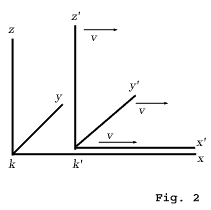Similarly, we can imagine the train travelling with the velocity v to be continued across the whole of space, so that every event, no matter how far off it may be, could also be localised with respect to the second framework. Without committing any fundamental error, we can disregard the fact that in reality these frameworks would continually interfere with each other, owing to the impenetrability of solid bodies. In every such framework we imagine three surfaces perpendicular to each other marked out, and designated as " co-ordinate planes " (" co-ordinate system "). A co-ordinate system K then corresponds to the embankment, and a co-ordinate system K' to the train. An event, wherever it may have taken place, would be fixed in space with respect to K by the three perpendiculars x, y, z on the co-ordinate planes, and with regard to time by a time value t. Relative to K1, the same event would be fixed in respect of space and time by corresponding values x1, y1, z1, t1, which of course are not identical with x, y, z, t. It has already been set forth in detail how these magnitudes are to be regarded as results of physical measurements.

Obviously our problem can be exactly formulated in the following manner. What are the values x1, y1, z1, t1, of an event with respect to K1, when the magnitudes x, y, z, t, of the same event with respect to K are given ? The relations must be so chosen that the law of the transmission of light in vacuo is satisfied for one and the same ray of light (and of course for every ray) with respect to K and K1. For the relative orientation in space of the co-ordinate systems indicated in the diagram (Fig. 2), this problem is solved by means of the equations :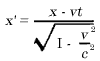y1 = y
z1 = z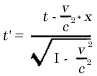This system of equations is known as the " Lorentz transformation." 1)

If in place of the law of transmission of light we had taken as our basis the tacit assumptions of the older mechanics as to the absolute character of times and lengths, then instead of the above we should have obtained the following equations:

x1 = x - vt
y1 = y
z1 = z
t1 = t

This system of equations is often termed the " Galilei transformation." The Galilei transformation can be obtained from the Lorentz transformation by substituting an infinitely large value for the velocity of light c in the latter transformation.

Aided by the following illustration, we can readily see that, in accordance with the Lorentz transformation, the law of the transmission of light in vacuo is satisfied both for the reference-body K and for the reference-body K1. A light-signal is sent along the positive x-axis, and this light-stimulus advances in accordance with the equation

x = ct,

i.e. with the velocity c. According to the equations of the Lorentz transformation, this simple relation between x and t involves a relation between x1 and t1. In point of fact, if we substitute for x the value ct in the first and fourth equations of the Lorentz transformation, we obtain: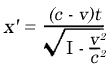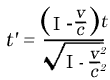from which, by division, the expression

x1 = ct1

immediately follows. If referred to the system K1, the propagation of light takes place according to this equation. We thus see that the velocity of transmission relative to the reference-body K1 is also equal to c. The same result is obtained for rays of light advancing in any other direction whatsoever. Of cause this is not surprising, since the equations of the Lorentz transformation were derived conformably to this point of view.

#### Footnotes

1) A simple derivation of the Lorentz transformation is given in Appendix I.